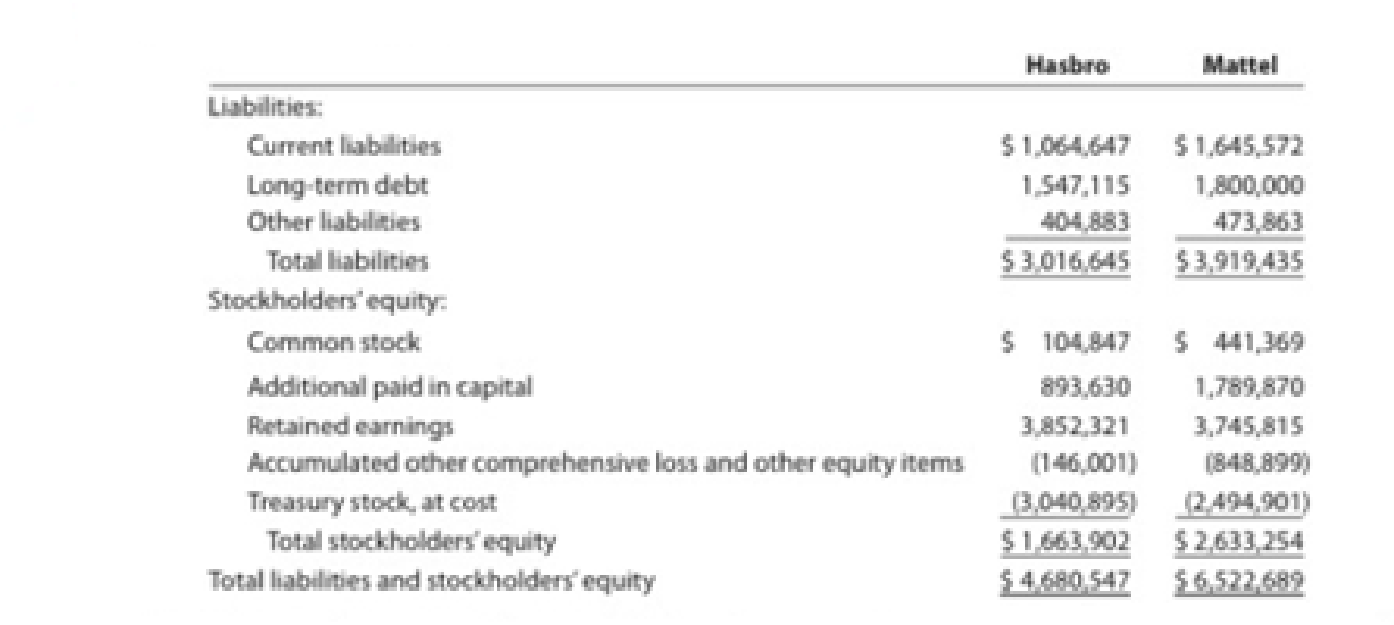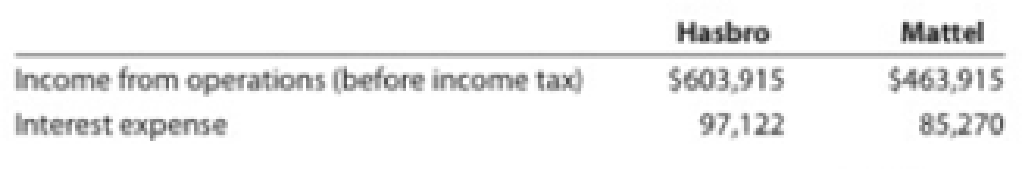Chapter 17, Problem 14E

Chapter
Section
Textbook Problem
1 views

# Ratio of liabilities to stockholders’ equity and times interest earnedHasbro, Inc. and Mattel, Inc. are the two largest toy companies in North America. Condensed liabilities and stockholders’ equity from a recent balance sheet are shown for each company as follows (in thousands):The income from operations and interest expense from the income statement for each company were as follows (in thousands):a. Determine the ratio of liabilities to stockholders’ equity for both companies. Round to one decimal place. b. Determine the times interest earned ratio for both companies. Round to one decimal place. c. Interpret the ratio differences between the two companies.

(a)

To determine

Compute ratio of liabilities to stockholders’ equity.

Explanation

Financial Ratios: Financial ratios are the metrics used to evaluate the liquidity, capabilities, profitability, and overall performance of a company.

Ratio of liabilities to stockholders’ equity is determined by dividing liabilities and stockholders’ equity.

Formula:

Ratio of liabilities to stockholders' equity=Total liabilitiesStockholders' equity

Compute ratio of liabilities to stockholders’ equity for H.

Ratio of liabilities to stockholders' equity}=Total liabilitiesStockholders' equity=

(b)

To determine

Compute times interest earned ratio.

(c)

To determine

Provide conclusion about company’s ability to meet its currently maturing debt.

### Still sussing out bartleby?

Check out a sample textbook solution.

See a sample solution

#### The Solution to Your Study Problems

Bartleby provides explanations to thousands of textbook problems written by our experts, many with advanced degrees!

Get Started